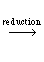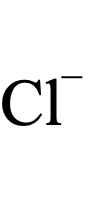Periodic TableT-Shirts & morefrom themeta-synthesis Merch Store# Redox Chemistry Synthlet

Synthlets are small software applications – applets – that use simple theories, models & techniques to predict the synthesis of small molecules.Predict the outcome of redox reactions with this Synthlet. Select oxidising agents and reducing agents from the drop boxes below:
 Oxidising Agent, Reduction Reaction         Reducing Agent, Oxidation Reaction Select Oxidising Agent F2   →   F–O3   →   O2Co3+   →   Co2+H2O2   →   H2ONO2   →   N2MnO4–   →   MnO2PbO2   →   Pb4+Au+   →   AuPb4+   →   Pb2+ClOH   →   Cl2BrOH   →   Br2MnO4–   →   Mn2+PbO2   →   Pb2+IO–   →   I2Au3+   →   Au+Cl2   →   Cl–Cr2O72–   →   Cr3+Ce4+   →   Ce3+Tl3+   →   Tl+MnO2   →   Mn2+O2   →   H2OIO3–   →   I2Pt2+   →   PtAg2O   →   AgBr2   →   Br–HNO2   →   NOPd2+   →   PdNO3–   →   NONO3–   →   HNO2Hg2+   →   Hg+NO3–   →   [NH4]+Hg2+   →   HgNO3–   →   N2O4Ag+   →   AgHg+   →   HgFe3+   →   Fe2+O2   →   H2O2I2   →   I–Cu2+   →   CuClCu+   →   CuH2SO3   →   SCd2+   →   CdO2   →   HO–Cu2+   →   CuHg2Cl2   →   HgPbO   →   PbGe2+   →   GeAgCl   →   AgSO4–   →   H2SO3S   →   H2SCu2+   →   Cu+Sn4+   →   Sn2+CuCl   →   CuSi   →   SiH4P   →   PH3H+   →   H2Fe3+   →   FeCO2   →   COPb2+   →   PbSn2+   →   SnV2+   →   VCO2   →   HCOOHIn+   →   InV3+   →   V2+Ni2+   →   NiCo2+   →   CoH3PO4   →   P(OH)3Tl+   →   TlPbSO4   →   PbSe   →   SeH2Ti3+   →   Ti2+Ga3+   →   Ga+In3+   →   In+Cr3+   →   Cr2+Fe2+   →   FeS   →   S2–CO2   →   (COOH)2P(OH)3   →   HP(OH)2Sb   →   SbH3HP(OH)2   →   PGa3+   →   GaAs   →   AsH3U4+   →   U3+Cr3+   →   CrZn2+   →   ZnSiO2   →   SiCr2+   →   CrSe   →   Se2–Te   →   Te2–Mn2+   →   MnTi2+   →   TiAl3+   →   AlU3+   →   UBe2+   →   BeMg2+   →   MgCe3+   →   CeLa3+   →   LaNa+   →   NaCa2+   →   CaSr2+   →   SrBa2+   →   BaCs+   →   CsRb+   →   RbK+   →   KLi+   →   LiN2   →   HN3                    Select Reducing Agent HN3   →   N2Li   →   Li+K   →   K+Cs   →   Cs+Rb   →   Rb+Ba   →   Ba2+Sr   →   Sr2+Ca   →   Ca2+Na   →   Na+La   →   La3+Ce   →   Ce3+Mg   →   Mg2+Be   →   Be2+U   →   U3+Al   →   Al3+Ti   →   Ti2+Mn   →   Mn2+Te2–   →   TeSe2–   →   SeCr   →   Cr2+Si   →   SiO2Zn   →   Zn2+Cr   →   Cr3+U3+   →   U4+AsH3   →   AsGa   →   Ga3+SbH3   →   SbP   →   HP(OH)2HP(OH)2   →   P(OH)3(COOH)2   →   CO2S2–   →   SFe   →   Fe2+Cr2+   →   Cr3+Ga+   →   Ga3+In+   →   In3+SeH2   →   SeTi2+   →   Ti3+Pb   →   PbSO4Tl   →   Tl+Co   →   Co2+P(OH)3   →   H3PO4Ni   →   Ni2+V2+   →   V3+In   →   In+HCOOH   →   CO2V   →   V2+Sn   →   Sn2+Pb   →   Pb2+CO   →   CO2Fe   →   Fe3+H2   →   H+PH3   →   PSiH4   →   SiCu   →   CuClSn2+   →   Sn4+Cu+   →   Cu2+H2SO3   →   SO4–H2S   →   SAg   →   AgClGe   →   Ge2+Pb   →   PbOHg   →   Hg2Cl2Cu   →   Cu2+Cd   →   Cd2+HO–   →   O2S   →   H2SO3Cu   →   Cu+CuCl   →   Cu2+I–   →   I2H2O2   →   O2Fe2+   →   Fe3+Hg   →   Hg+N2O4   →   NO3–Ag   →   Ag+Hg   →   Hg2+ [NH4]+   →   NO3–Hg+   →   Hg2+HNO2   →   NO3–NO   →   NO3–Pd   →   Pd2+NO   →   HNO2Br–   →   Br2Ag   →   Ag2OI2   →   IO3–Pt   →   Pt2+Mn2+   →   MnO2H2O   →   O2Tl+   →   Tl3+Ce3+   →   Ce4+Cr3+   →   Cr2O72–Cl–   →   Cl2Au+   →   Au3+I2   →   IO–Pb2+   →   PbO2Mn2+   →   MnO4–Br2   →   BrOHCl2   →   ClOHPb2+   →   Pb4+Au   →   Au+Pb4+   →   PbO2MnO2   →   MnO4–H2O   →   H2O2N2   →   NO2Co2+   →   Co3+O2   →   O3F–   →   F2                    & Help
Oxidising agent gains electron(s): reduction reaction    Cl2   →   Cl   E° = +1.36
 1+ 22Reducing agent releases electron(s): oxidation reaction    Na   →   Na+   E° = -2.71
 11+ 1The Galvanic Cell Wikipedia Rule: The reaction will proceed in a forward direction, from left to right, if the cell potential E°cell is net positive. E° reduction rxn – E° oxidation rxn = E° cell +1.36 – -2.71 = +4.07||||ReducingAgent OxidisedSpecies OxidisingAgent ReducedSpecies
 2+ 12+ 2Sodium Sodium ion Chlorine Chloride ion
Sodium, the reducing agent, is oxidised to Sodium ion by Chlorine
and Chlorine, the oxidising agent, is reduced to Chloride ion by Sodium.

© Mark R. Leach 1999 –

If you have any:

Queries